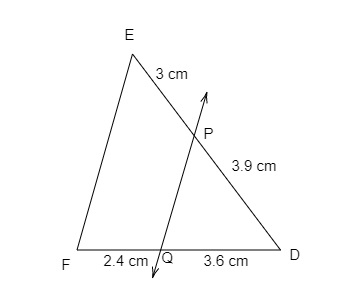"
">

# In the figure below, state if $PQ\parallel EF$."

Given:

$DP=3.9\ cm, PE=3\ cm, DQ=3.6\ cm$ and $QF=2.4\ cm$.
To do:

We have to find if $PQ\parallel EF$.

Solution:

We know that,

If a line divides two sides of a triangle proportionally, then it is parallel to the third side.
Therefore,

$\frac{DP}{PE}=\frac{3.9}{3}=1.3$

$\frac{DQ}{QF}=\frac{3.6}{2.4}=\frac{3}{2}=1.5$
$\frac{DP}{PE}≠\frac{DQ}{QF}$

Hence, $PQ$ is not parallel to $EF$.

Updated on: 10-Oct-2022

21 Views